Hits: 4

# Bellman Ford’s Algorithm

#### Bellman Ford algorithm helps us find the shortest path from a vertex to all other vertices of a weighted graph.

It is similar to Dijkstra’s algorithm but it can work with graphs in which edges can have negative weights.

## Why would one ever have edges with negative weights in real life?

Negative weight edges might seem useless at first but they can explain a lot of phenomena like cashflow, the heat released/absorbed in a chemical reaction, etc.

For instance, if there are different ways to reach from one chemical A to another chemical B, each method will have sub-reactions involving both heat dissipation and absorption.

If we want to find the set of reactions where minimum energy is required, then we will need to be able to factor in the heat absorption as negative weights and heat dissipation as positive weights.

## Why do we need to be careful with negative weights?

Negative weight edges can create negative weight cycles i.e. a cycle that will reduce the total path distance by coming back to the same point.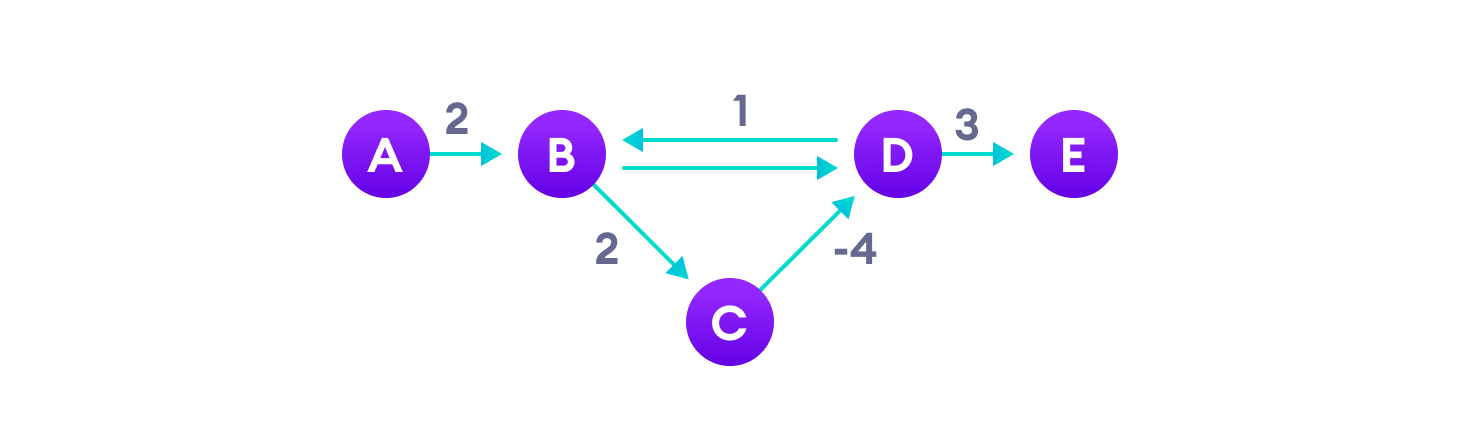Negative weight cycles can give an incorrect result when trying to find out the shortest path

Shortest path algorithms like Dijkstra’s Algorithm that aren’t able to detect such a cycle can give an incorrect result because they can go through a negative weight cycle and reduce the path length.

## How Bellman Ford’s algorithm works

Bellman Ford algorithm works by overestimating the length of the path from the starting vertex to all other vertices. Then it iteratively relaxes those estimates by finding new paths that are shorter than the previously overestimated paths.

By doing this repeatedly for all vertices, we can guarantee that the result is optimized.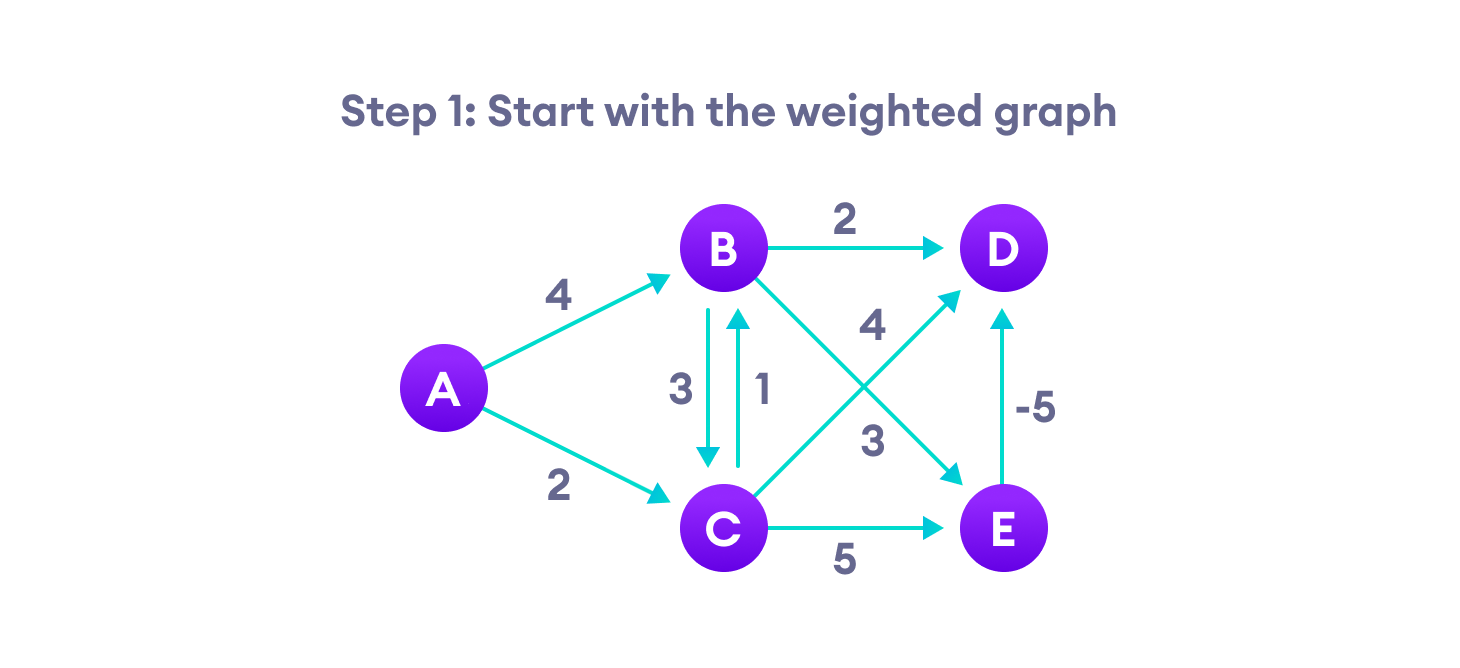Step-1 for Bellman Ford’s algorithm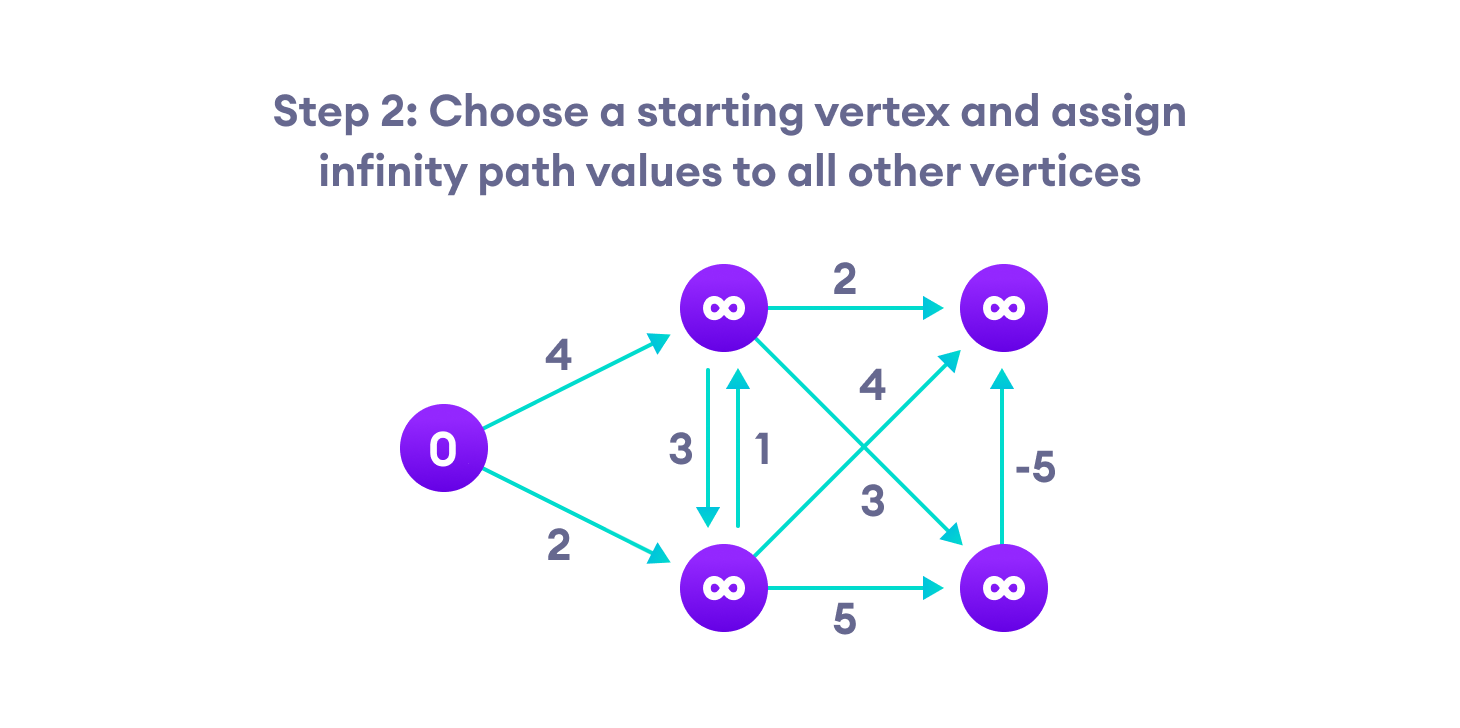Step-2 for Bellman Ford’s algorithm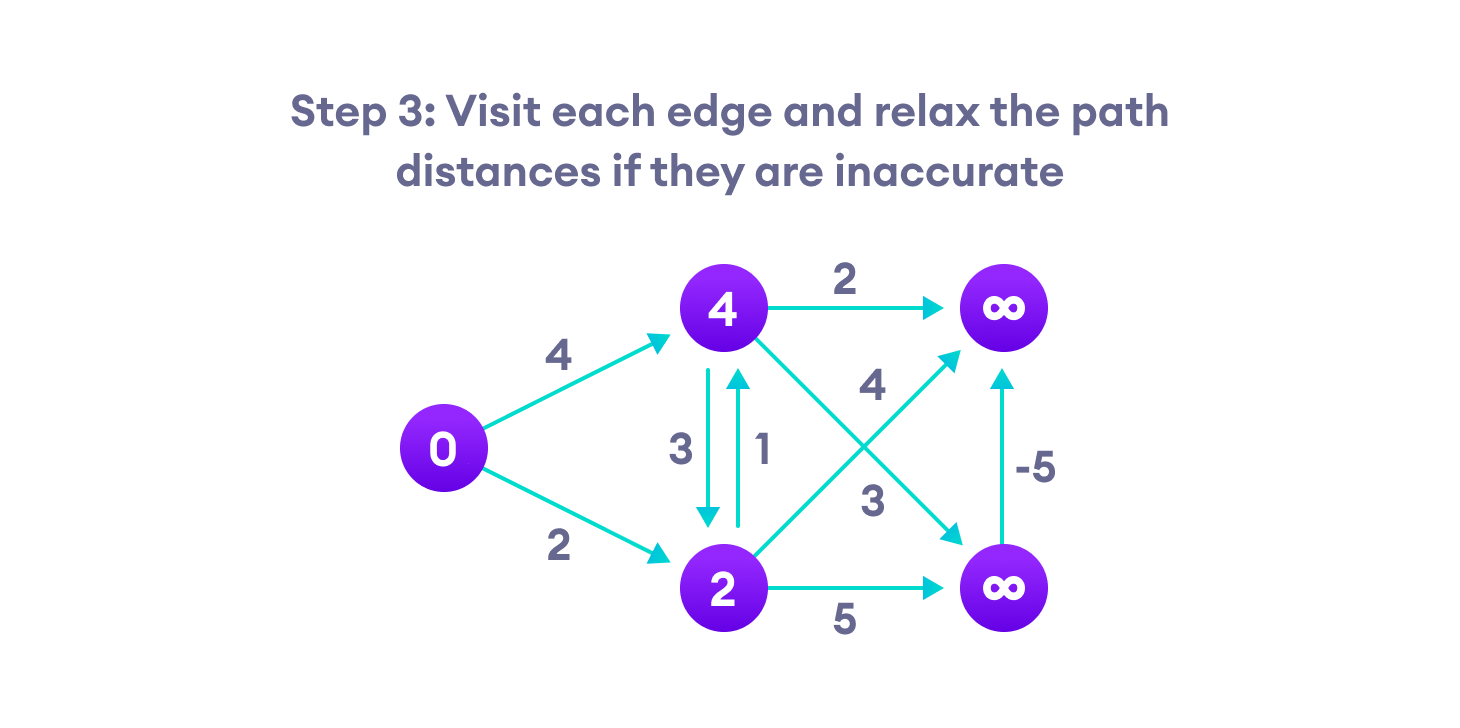Step-3 for Bellman Ford’s algorithmStep-4 for Bellman Ford’s algorithm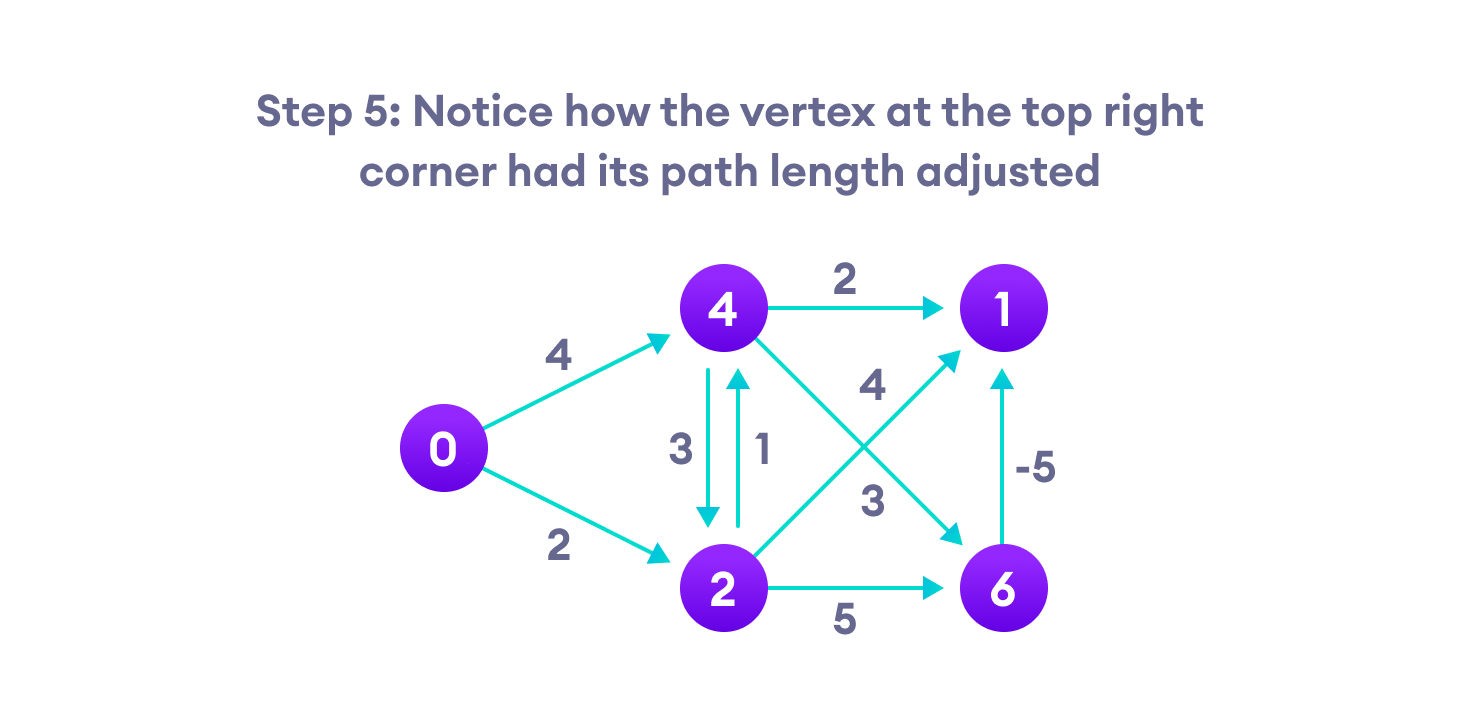Step-5 for Bellman Ford’s algorithm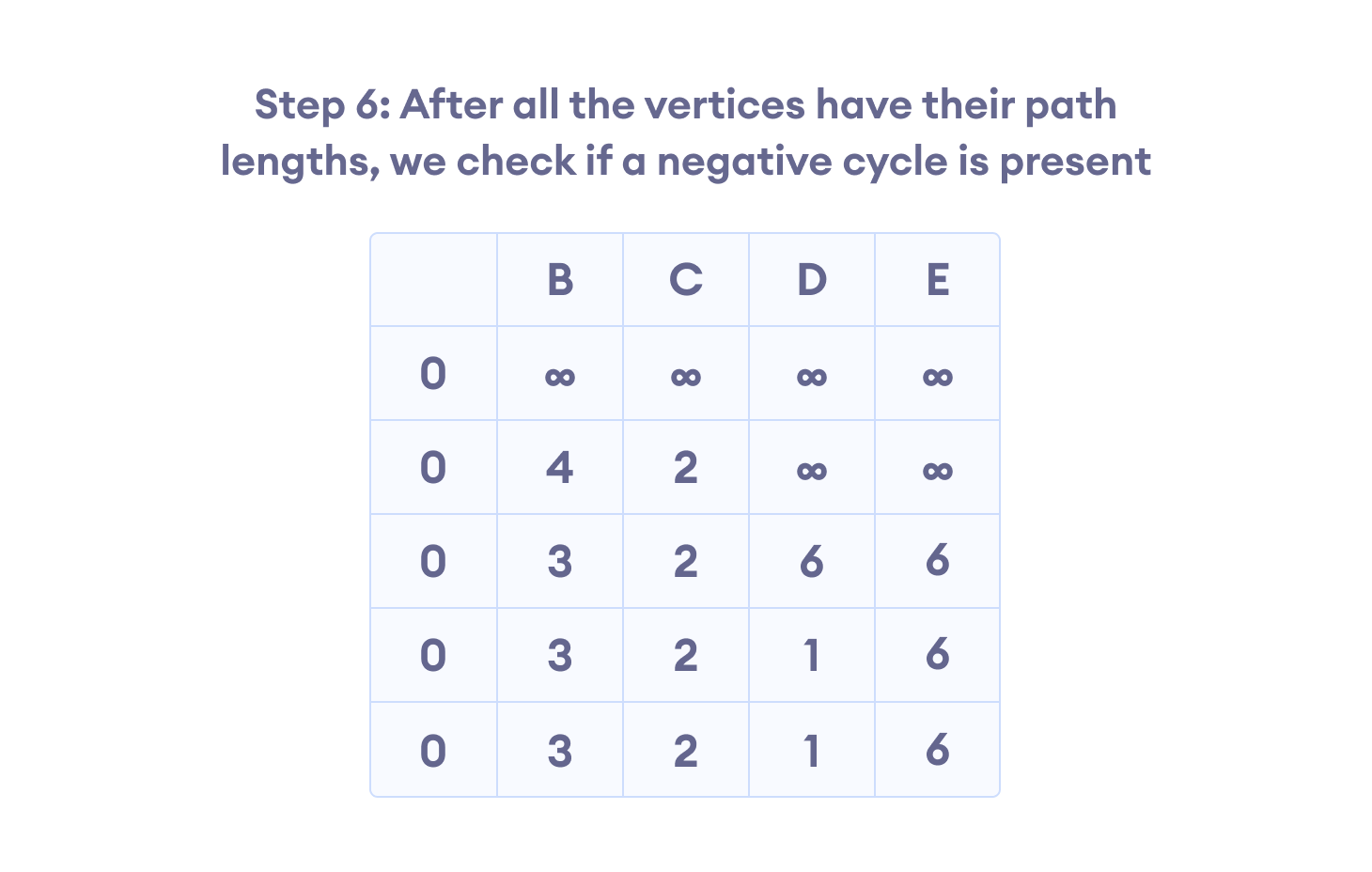Step-6 for Bellman Ford’s algorithm

## Bellman Ford Pseudocode

We need to maintain the path distance of every vertex. We can store that in an array of size v, where v is the number of vertices.

We also want to be able to get the shortest path, not only know the length of the shortest path. For this, we map each vertex to the vertex that last updated its path length.

Once the algorithm is over, we can backtrack from the destination vertex to the source vertex to find the path.

```function bellmanFord(G, S)
for each vertex V in G
distance[V] <- infinite
previous[V] <- NULL
distance[S] <- 0

for each vertex V in G
for each edge (U,V) in G
tempDistance <- distance[U] + edge_weight(U, V)
if tempDistance < distance[V]
distance[V] <- tempDistance
previous[V] <- U

for each edge (U,V) in G
If distance[U] + edge_weight(U, V) < distance[V}
Error: Negative Cycle Exists

return distance[], previous[]```

## Bellman Ford vs Dijkstra

Bellman Ford’s algorithm and Dijkstra’s algorithm are very similar in structure. While Dijkstra looks only to the immediate neighbors of a vertex, Bellman goes through each edge in every iteration.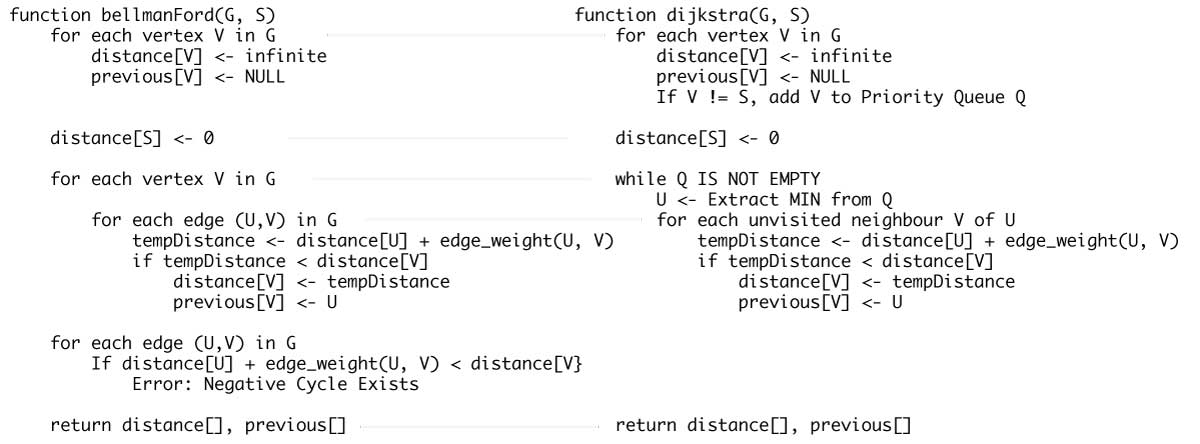Dijkstra’s vs Bellman Ford’s Algorithm

## Python Examples

``````/* Bellman Ford Algorithm in Python */

class Graph:

def __init__(self, vertices):
self.V = vertices   /* Total number of vertices in the graph */
self.graph = []     /* Array of edges */

/* Add edges */
def add_edge(self, s, d, w):
self.graph.append([s, d, w])

/* Print the solution */
def print_solution(self, dist):
print("Vertex Distance from Source")
for i in range(self.V):
print("{0}tt{1}".format(i, dist[i]))

def bellman_ford(self, src):

/* Step 1: fill the distance array and predecessor array */
dist = [float("Inf")] * self.V
/* Mark the source vertex */
dist[src] = 0

/* Step 2: relax edges |V| - 1 times */
for _ in range(self.V - 1):
for s, d, w in self.graph:
if dist[s] != float("Inf") and dist[s] + w < dist[d]:
dist[d] = dist[s] + w

/* Step 3: detect negative cycle
if value changes then we have a negative cycle in the graph
and we cannot find the shortest distances */
for s, d, w in self.graph:
if dist[s] != float("Inf") and dist[s] + w < dist[d]:
print("Graph contains negative weight cycle")
return

/* No negative weight cycle found!
Print the distance and predecessor array */
self.print_solution(dist)

g = Graph(5)
g.add_edge(0, 1, 5)
g.add_edge(0, 2, 4)
g.add_edge(1, 3, 3)
g.add_edge(2, 1, 6)
g.add_edge(3, 2, 2)

g.bellman_ford(0)``````

## Bellman Ford’s Complexity

### Time Complexity

 Best Case Complexity O(E) Average Case Complexity O(VE) Worst Case Complexity O(VE)

### Space Complexity

And, the space complexity is `O(V)`.

## Bellman Ford’s Algorithm Applications

1. For calculating shortest paths in routing algorithms
2. For finding the shortest path

# Special 95% discount

## 2000+ Applied Machine Learning & Data Science Recipes

### Portfolio Projects for Aspiring Data Scientists: Tabular Text & Image Data Analytics as well as Time Series Forecasting in Python & R## Two Machine Learning Fields

There are two sides to machine learning:

• Practical Machine Learning:This is about querying databases, cleaning data, writing scripts to transform data and gluing algorithm and libraries together and writing custom code to squeeze reliable answers from data to satisfy difficult and ill defined questions. It’s the mess of reality.
• Theoretical Machine Learning: This is about math and abstraction and idealized scenarios and limits and beauty and informing what is possible. It is a whole lot neater and cleaner and removed from the mess of reality.

Data Science Resources: Data Science Recipes and Applied Machine Learning Recipes

Introduction to Applied Machine Learning & Data Science for Beginners, Business Analysts, Students, Researchers and Freelancers with Python & R Codes @ Western Australian Center for Applied Machine Learning & Data Science (WACAMLDS) !!!

Latest end-to-end Learn by Coding Recipes in Project-Based Learning:

Applied Statistics with R for Beginners and Business Professionals

Data Science and Machine Learning Projects in Python: Tabular Data Analytics

Data Science and Machine Learning Projects in R: Tabular Data Analytics

Python Machine Learning & Data Science Recipes: Learn by Coding

R Machine Learning & Data Science Recipes: Learn by Coding

Comparing Different Machine Learning Algorithms in Python for Classification (FREE)

`Disclaimer: The information and code presented within this recipe/tutorial is only for educational and coaching purposes for beginners and developers. Anyone can practice and apply the recipe/tutorial presented here, but the reader is taking full responsibility for his/her actions. The author (content curator) of this recipe (code / program) has made every effort to ensure the accuracy of the information was correct at time of publication. The author (content curator) does not assume and hereby disclaims any liability to any party for any loss, damage, or disruption caused by errors or omissions, whether such errors or omissions result from accident, negligence, or any other cause. The information presented here could also be found in public knowledge domains.  `